## Determinant

Each n by n square matrix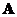has an associated scalar number, we called as determinant of that matrix, whose value will determine whether the matrix has an inverse or not. The symbol of determinant is either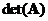or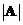.

When matrix order is 1,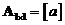then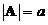When the matrix order is 2,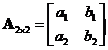, then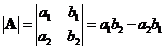When the matrix order is 3,, thenWhen we have a matrix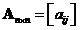then we can define several things:

1. The minor of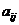is the determinant of a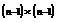sub matrix denoted by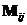. The sub matrixobtained from matrix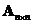by deleting the row and column containing.
Example: we have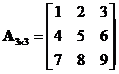then the sub matrix of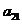is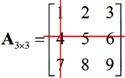or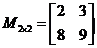and the minor is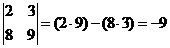2. The signed minor is called cofactor ofdenoted by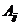. Cofactor is a scalar sign determined by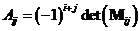. This sign follows the following order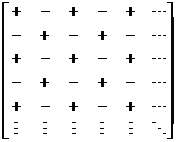Example:

Suppose we havethen the cofactor ofis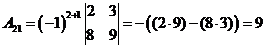The determinant of matrixis the sum product of the first column entries with its cofactor, that is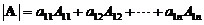For example,, then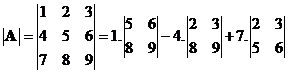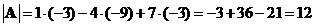Hand (manual) computation of determinant of matrix size larger than 3 is quite time consuming. The interactive program below will help you to compute determinant of your square matrix of any positive order less than 10. Random Example button will generate random square matrix of random size.

## Properties

The following are well known properties of matrix determinant:

• A square matrixis singular (has no inverse) if and only if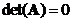• If a square matrixhas an inverse, the determinant of an inverse matrix is the reciprocal of the matrix determinant, that is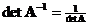.
• When matrixhas a row or a column consisting entirely of zeros, then• When matrixhas two identical rows or two identical columns, then• Determinant of a matrix product is equal to the product of their determinant,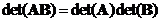• Determinant does not change when we transpose the matrix,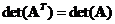• Determinant does not change when we add a multiple of a row or a column to another.
• Interchanging two rows or two columns of a matrix changes the sign of the determinant but the magnitude of the determinant does not change.
• Multiplying a row or a column of a matrix by a scalar will make the value of the determinant multiplied by that scalar. Similarly, removing a common factor from a row or a column will also remove the common factor from its determinant.
• When matrixis an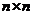triangular matrix, the determinant equals the product of the diagonal elements of